# Test: Trigonometry- 1

## 10 Questions MCQ Test Quantitative Reasoning for GMAT | Test: Trigonometry- 1

Description
Attempt Test: Trigonometry- 1 | 10 questions in 20 minutes | Mock test for GMAT preparation | Free important questions MCQ to study Quantitative Reasoning for GMAT for GMAT Exam | Download free PDF with solutions
QUESTION: 1

### A 60 feet long ladder is inclined against a wall such that the bottom and the top of the ladder are at equal distance from the line where the wall meets the floor. The same ladder is then moved to a new position such that it is inclined at an angle of 30o from the floor. What is the difference between the heights to which the ladder reaches in the two cases?

Solution:

Step 1: Question statement and Inferences

Let us represent the two cases diagrammatically.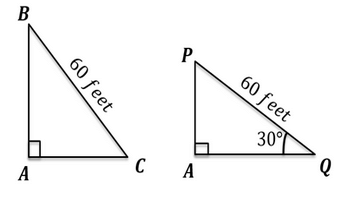Left Triangle BAC represents the initial case. Point A is on the line of intersection of the wall and the floor.

We are given that AB = AC and that BC = 60 feet.

Right Triangle PAQ represents the case after the ladder is moved. The only value that remains unchanged after the movement is the ladder’s length. Hence, in Right Triangle PAQ,

PQ = 60 feet, and Angle AQP = 30°

We need to find the value of AB – AP.

Step 2: Finding required values

Let us first consider Right Triangle BAC:

We know that when two sides of a right triangle are equal, it is a 45°-45°-90° triangle.

In such a triangle, the sides opposite to the angles 45°, 45°, and 90° respectively are in the ratio 1 : 1 : √2

Therefore,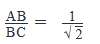Or,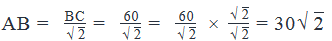Thus, we have found out the value of AB.

Now, considering Right Triangle PAQ:

It is a 30°-60°-90° right angled triangle.

We know that in such a triangle, the sides opposite to the angles 30°, 60°, and 90° respectively are in  the ratio 1 : √3 : 2.

Therefore,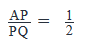or,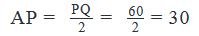Step 3: Calculate the final answer

Difference between the heights to which the ladder reaches in the two cases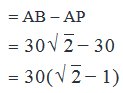QUESTION: 2

###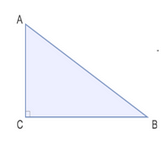In the given figure, AB is a ladder inclined at an angle of 30o above the ground. If the length of the ladder is x feet, the ladder reaches z feet above the ground and the bottom of the ladder is y feet from the wall, what is the value of the sum x+y+z? (1)  The ratio of the lengths of sides AB:BC:AC is 2: √3 : 1 (2)  The length of the ladder is 12 feet

Solution:

We are given that                               AngleABC=30∘

△ABC   is a right-triangle (as indicated in the given figure)

Therefore, from the Angle Sum Property:

Angle ABC+ Angle BCA+ Angle CAB = 180

30 +90+ AngleCAB =180

Thus,

AngleCAB=60

Therefore, △ABC  is a 30°-60°-90° right angled triangle. In other words, the ladder forms a 30°-60°-90° right angled triangle with the wall.

We know that in such a triangle, the sides opposite to the angles 30°, 60°, and 90° respectively are in the ratio 1 : √3 : 2.

Thus, z : y : x = 1 : √3 : 2

This implies, y = z√3

And, x = 2z

So, the sum x + y + z = 2z + z√3 + z

x + y + z = z(3+√3)

Thus, in order to find the value of the sum, we need to know the value of z or of any other side of the triangle ABC.

Step 3: Analyze Statement 1

The ratio of sides AB:BC:AC is 2: 3 : 1

We are given the ratio of sides of triangle. We have already deduced this ratio of sides in Steps 1 and 2. So Statement 1 gives us no new information.

This question illustrates well the importance of first extracting the maximum juice from the question statement before moving to Statement 1. A student who skips doing this and straightaway jumps to Statement 1 runs the risk of choosing (C) as the answer in this question.

Statement 1 is clearly Not Sufficient.

Step 4: Analyze Statement 2

The length of the ladder is 12 feet.

This means, x = 12 feet

We also know that x = 2z and y = z√3

Hence, we can easily find out the values of y and z.

Thus Statement 2 alone is Sufficient.

Step 5: Analyze Both Statements Together (if needed)

We get a unique answer in step 4, so this step is not required

QUESTION: 3

### A crane is trying to reach the ninth floor of an under-construction multi-storey building. The length of the crane’s arm is flexible and can be increased or decreased. To reach the ninth floor, the crane raises its arm at an elevation angle of 30o and the arm length is 180 feet. The crane then moves closer to the building to reach the same floor at a different elevation angle. How much distance did the crane move? Assume the crane’s height to be negligible. (1). After the movement, the elevation angle of the crane's arm is 60o. (2). After the movement, the crane is at a distance of 30√3 feet from the building.

Solution:

Steps 1 & 2: Understand Question and Draw Inferences

The flexibility of the crane’s arm is an important point here. Remember that the ninth floor is at a fixed height. Hence when the crane moves closer to the building, its elevation angle will increase for sure and its arm length will decrease. Hence the only constant here is the height of the ninth floor (from the ground).

Hence, in order to find out the distance moved by the crane, we first need to find the constant height.

Let’s represent the given information diagrammatically.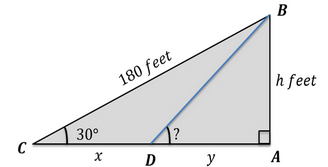In right triangle ABC, AB represents the building till the ninth floor. The length of AB is assumed to be h feet. BC represents the arm of the crane initially, when the angle of elevation was 30. From the question statement, we know that BC = 180 feet.

Since the crane’s height is given to be negligible, we have shown the arm of the crane as starting from the ground level only. Thus, points C and A lie on the horizontal ground, and the line AC is therefore perpendicular to the building AB.

Right triangle BAC is a 30°-60°-90° triangle.

We know that in such a triangle, the sides opposite to the angles 30°, 60°, and 90° respectively are in  the ratio 1 : √3 : 2.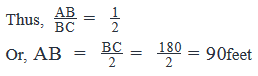So, h = 90 feet.

Also,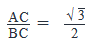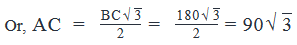Let the crane move x feet towards the building to point D.

Let the distance AD = y feet.

Thus, x + y = 90√3

So, if we are able to find the value of y, we will be able to find the value of x.

We have only one piece of information about right triangle DAB, that AB = 90 feet.

If we get one more piece of information about this triangle, we will be able to find the value of y.

Thus, in order to find the value of y, we need to know either one of the two unknown sides or one of the two unknown angles of Triangle DAB.

Step 3: Analyze Statement 1

After the movement, the elevation angle of the crane’s arm is 60

This means that Triangle DAB is a 30°-60°-90° triangle.

We know that in such a triangle, the sides opposite to the angles 30°, 60°, and 90° respectively are in  the ratio 1 : √3 : 2.

Thus, y =  30√3

Since we know the value of y, we will be able to find the value of x.

Thus, Statement 1 alone is sufficient to answer the question.

(Note: We have shown the full calculation here just to demonstrate how the value of y may be calculated, if a PS question was made on the same situation. Since this is a Data Sufficiency question, you need not actually solve till the value of y. Once you analyze that the given data is sufficient to find the value of y, you can move on to Step 4)

Step 4: Analyze Statement 2

After the movement, the crane is at a distance of 30√3 feet from the building.

That is, y = 30√3

Since we know the value of y, we will be able to calculate the value of x.

Thus, Statement 2 is Sufficient.

Step 5: Analyze Both Statements Together (if needed)

We get a unique answer in steps 3 and 4, so this step is not required

QUESTION: 4

Triangle ACB is right angled at C . The perpendicular drawn from point C on the side AB bisects AB at point D . If CD = 5 cm, what is the area of Triangle ACB?

Solution:

In order to make sense of the given information, let’s depict it visually: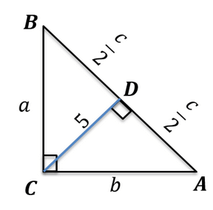We have assumed the lengths of sides BC, AC and AB to be a, b and c cm respectively.

CD is the perpendicular drawn from point C on the side AB.

We need to find out area of triangle ACB. We know that,

Area of a right angled triangle =1/2 x base x height

So, Area of triangle ACB =1/2 × AC × BC = 1/2 × AB × CD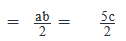....Equation 1

Thus, in order to calculate the area of DACB, we need to know either the values of a and b or the value of c.

Step 2: Finding required values

In Right Triangle ACB,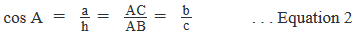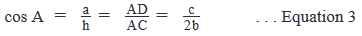By comparing Equations 2 and 3, we get: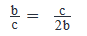This simplifies to:

c2=2b2

Or,

c=√2b                                   . . . Equation 4

In Right Triangle ACB,

By Pythagoras Theorem:

a2+b2=c2

Substituting Equation 4 in this equation, we get:

a2+b2=2b2

Or,

a2=b2

Which means, a = b

Since two sides of right triangle ACB are equal, this means it is a 45°-45°-90° triangle.

Therefore, Angle CAB = Angle ABC = 45°

Right Triangle ADC too will therefore be a 45°-45°-90° triangle.

Therefore, in right triangle ADC, the sides opposite to the angles 45°, 45°, and 90° respectively are in the ratio 1 : 1 : √2

So, AD : CD : AC =  1 : 1 : √2       . . . Equation 5

Step 3: Calculate the final answer

From Equation 5,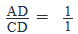Or, AD = CD = 5

c/2=5

Thus, c=10

From Equation 1,

Area of triangle ACB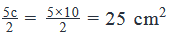QUESTION: 5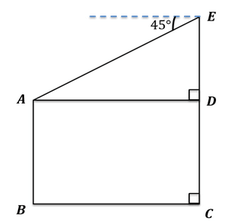In the figure above, lines AB and CE represent the two military posts guarding an area. From the top of the military post CE, the angle of depression of the top of the post AB is observed to be 45°.  What is the horizontal distance between the two posts?

(1)  The height of the post CE is 10 feet

(2)  The post CE is taller than the post AB by 3 feet

Solution:

Steps 1 & 2: Understand Question and Draw Inferences

Horizontal distance between the two posts = BC = AD

Thus, the question wants us to find the length of BC or AD.

Also, since the angle of depression from point E to point A is given to be 45°, this means that Angle EAD = 45°

Let’s represent this information in the diagram: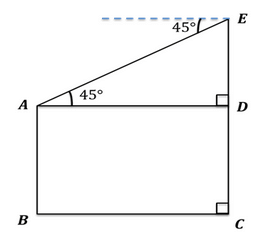We know that if one of the angles of a right triangle is 45°, then the third angle would be 45° too.

Also, we know that in such a triangle, the sides opposite to the angles 45°, 45°, and 90° respectively are in the ratio 1 : 1 : √2

AD : ED : AE =  1 : 1 : √2  . . . Let’s call this Equation 1.

From Equation 1 it is clear that to find the length of AD, we need to know the value of ED or AE.

Step 3: Analyze Statement 1

The height of the post CE is 10 feet

Thus, DC + ED = 10 . . . Equation 2

We need to know the value of ED.

But Equation 2 is a linear equation with 2 unknowns (DC and ED). Therefore, we will not be able to find a unique value of ED.

Thus Statement 1 alone is not sufficient.

Step 4: Analyze Statement 2

The post CE is taller than the post AB by 3 feet

Thus, CE – AB =3

Or, ED=3

Putting this value of ED in Equation 1, we will be able to find the value of AD.

Thus, Statement 2 alone is sufficient to arrive at a unique answer.

Step 5: Analyze Both Statements Together (if needed)

We get a unique answer in step 4, so this step is not required

QUESTION: 6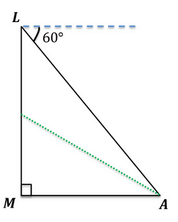In the figure above, LM is a lighthouse from the top of which John views object A at an angle of depression of 60o.

He then goes down the stairs to another spot in the middle of the lighthouse and views the same object at a different angle of depression. What is the distance between the bottom of the lighthouse and object A?

(1) The distance between the top of the lighthouse and object A is 80 feet.

(2) The distance between the middle of the lighthouse and object A is 20√7 feet.

Solution:

Steps 1 & 2: Understand Question and Draw Inferences

In this question, the angle between the horizontal and John’s line of sight when he looks at object A from point L is given to be 60o.

This means, in right triangle LMA, Angle MAL = 60°

By using the Angle Sum Property, we can find that Angle ALM = 30°

Triangle AML is a 30° -60° -90° triangle. In such a triangle, the sides opposite to the angles 30°, 60°, and 90° respectively are in the ratio 1 : √3 : 2

That is, MA: LM : AL = 1 : √3 : 2

Now, we need to find the distance between the bottom of the lighthouse and object A. That is, we need to find the length of MA.

Let the length of MA be x feet.

Thus,LM=3√x

And, AL=2x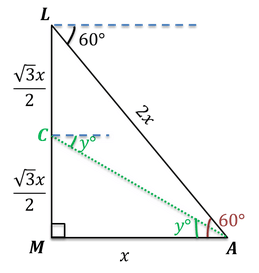John then moves downstairs to the middle of the lighthouse. Let the middle point be C.

So, MC=LC=√3x/2

Let the angle of depression from Point C to object A be y°

By Applying Pythagoras Theorem in right triangle AMC, we get:

AC2=MC2+AM2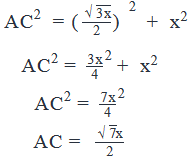Thus, we have expressed all sides of the right triangles AML and AMC in terms of x. If we know any of these sides, we will be able to find the value of x.

Step 3: Analyze Statement 1

The distance between the top of the lighthouse and object A is 80 feet

AL=80

This means

2x=80

x=40

Thus, Statement 1 alone is sufficient.

Step 4: Analyze Statement 2

The distance between the middle of the lighthouse and object A is 20√7 feet

AC=20√7

This means,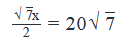x=40

Thus, Statement 2 alone is sufficient.

Step 5: Analyze Both Statements Together (if needed)

There is no need to analyze further as both statements (1) and (2) are sufficient.

QUESTION: 7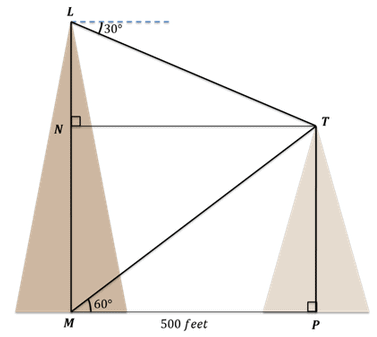The illustration depicts two hills of different heights LM and TP. When the top T of the second hill is viewed from the top L of the first hill, the angle of depression is 30°. When the top T is viewed from the foot M of the first hill, the angle of elevation is 60°. If the distance between points M and P is 500 feet, what is the difference between the heights of the two hills?

Solution:

Step 1: Question statement and Inferences

The question asks us to find the length of NL.

Let NL = x feet

We are given that the angle between the horizontal and a person’s line of sight when he looks at point T from point L is 30o.

This means, in right triangle LNT, Angle NTL =  30∘

Let’s depict this information in the figure: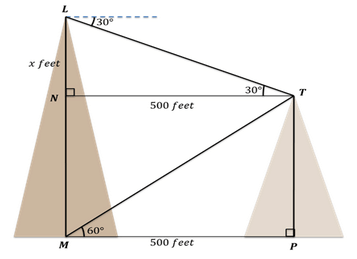Step 2: Finding required values

By applying the Angle Sum Property in right triangle LNT, we get

Angle TLN = 60°

Thus Triangle LNT is a 30°-60°-90° triangle. In a 30°-60°-90° triangle, the sides opposite to the angles 30°, 60°, and 90° respectively are in the ratio 1 : √3 : 2

That is, LN: NT : TL = 1 : √3 : 2

Also, in quadrilateral MPTN, lines MN and PT are both drawn perpendicular to the same line MP. Therefore, lines MN and PT are parallel to one another. Also, line NT is perpendicular to the vertical line MN; therefore, line NT is parallel to the horizontal line MP.

Thus, quadrilateral MPTN is made up of two sets of parallel lines and the enclosed angle is 90°

This means, quadrilateral MPTN is a rectangle.

Now, the opposite sides of a rectangle are equal.

Therefore, NT = MP = 500 feet

Step 3: Calculating the final answer

Therefore,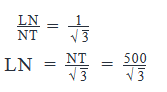Hence, the correct answer is Option A

QUESTION: 8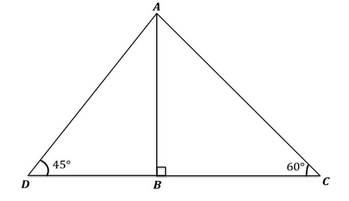Consider two triangles ABC and ABD with internal angles as shown and a common side AB. What is the length of side AC?

(2)   CD=10(√3+1)

Solution:

Steps 1 & 2: Understand Question and Draw Inferences

Here we can see that triangles ABC and ABD form the special triangles 30°-60°-90° and 45°-45°-90° respectively.

And we know that:

i)      In a 30°-60°-90° triangle, the sides opposite to the angles 30°, 60°, and 90° respectively are in the ratio 1 : √3 : 2

ii)     In a 45°-45°-90° triangle, the sides opposite to the angles 45°, 45°, and 90° respectively are in the ratio 1 : 1: √2

Let’s assume the length of side BC to be x units.

The lengths of all the other sides of the two triangles can be calculated in terms of x by applying the above ratios. The result will be as shown: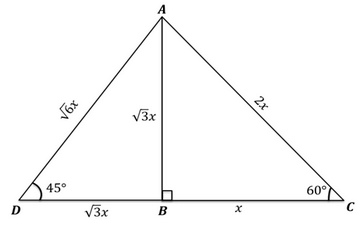Thus, in order to find the length of AC, we need to find the value of x.

Step 3: Analyze Statement 1

This means

(√6x)2+(2x)2=1000

6x2+4x2=1000

10x2=1000

x2=100

x=10

(The negative value of x is rejected because x, being the length of a geometrical figure, cannot be negative)

Since we know the value of x, we will be able to find the length of AC.

Thus, Statement 1 alone is sufficient.

Step 4: Analyze Statement 2

CD=10(√3+1)

This means,

x+3√x=10(3√+1)

x(1+√3)=10(√3+1)

x=10

Since we know the value of x, we will be able to find the length of AC.

Thus, Statement 2 alone is sufficient

Step 5: Analyze Both Statements Together (if needed)

We do not need to analyze further as both statements (1) and (2) are sufficient.

QUESTION: 9

3sinx + 4cosx + r is always greater than or equal to 0. What is the smallest value ‘r’ can to take?

Solution:

aSinx + bcosx can take a range of values. What is the possible range in this scenario?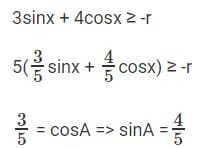5(sinx cosA + sinA cosx) ≥ -r
5(sin(x + A)) ≥ -r
5sin (x + A) ≥ -r
-1 ≤ sin (angle) ≤ 1
5sin (x + A) ≥ -5
rmin = 5
The question is "What is the smallest value ‘r’ can to take?"

QUESTION: 10

Sin2014x + Cos2014x = 1, x in the range of [-5π, 5π], how many values can x take?

Solution:

Number x lies between 0 and 1, higher powers of the number will be smaller than lower powers. Think about that. And then think about what does this have to do with Trigonometry.

We know that Sin2x + Cos2x = 1 for all values of x.
If Sin x or Cos x is equal to –1 or 1, then Sin2014x + Cos2014x will be equal to 1.

Sin x is equal to –1 or 1 when x = –4.5π or –3.5π or –2.5π or –1.5π or –0.5π or 0.5π or 1.5π or 2.5π or 3.5π or 4.5π.

Cosx is equal to –1 or 1 when x = –5π or –4π or –3π or –2π or –π or 0 or π or 2π or 3π or 4π or 5π.

For all other values of x, Sin2014 x will be strictly lesser than Sin2x.
For all other values of x, Cos2014 x will be strictly lesser than Cos2x.

We know that Sin2x + Cos2x is equal to 1. Hence, Sin2014x + Cos2014x will never be equal to 1 for all other values of x. Thus there are 21 values.

The question is "How many values the 'x' can take?"Use Code STAYHOME200 and get INR 200 additional OFF Use Coupon Code